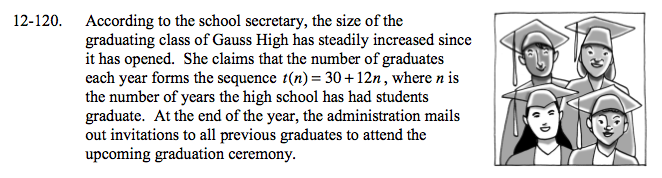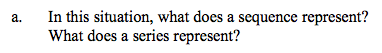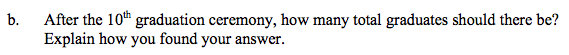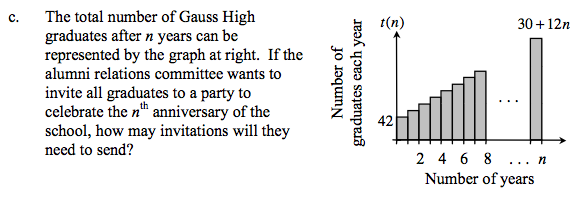### Home > A2C > Chapter 12 > Lesson 12.3.1 > Problem12-120

12-120.Remember that a sequence is a set of discrete numbers while a series is the sum of those numbers.$\text{Use the summation formula }S_{10}=\frac{10}{2}\left(t\left(1\right) + t\left(10\right)\right)$See part (b).